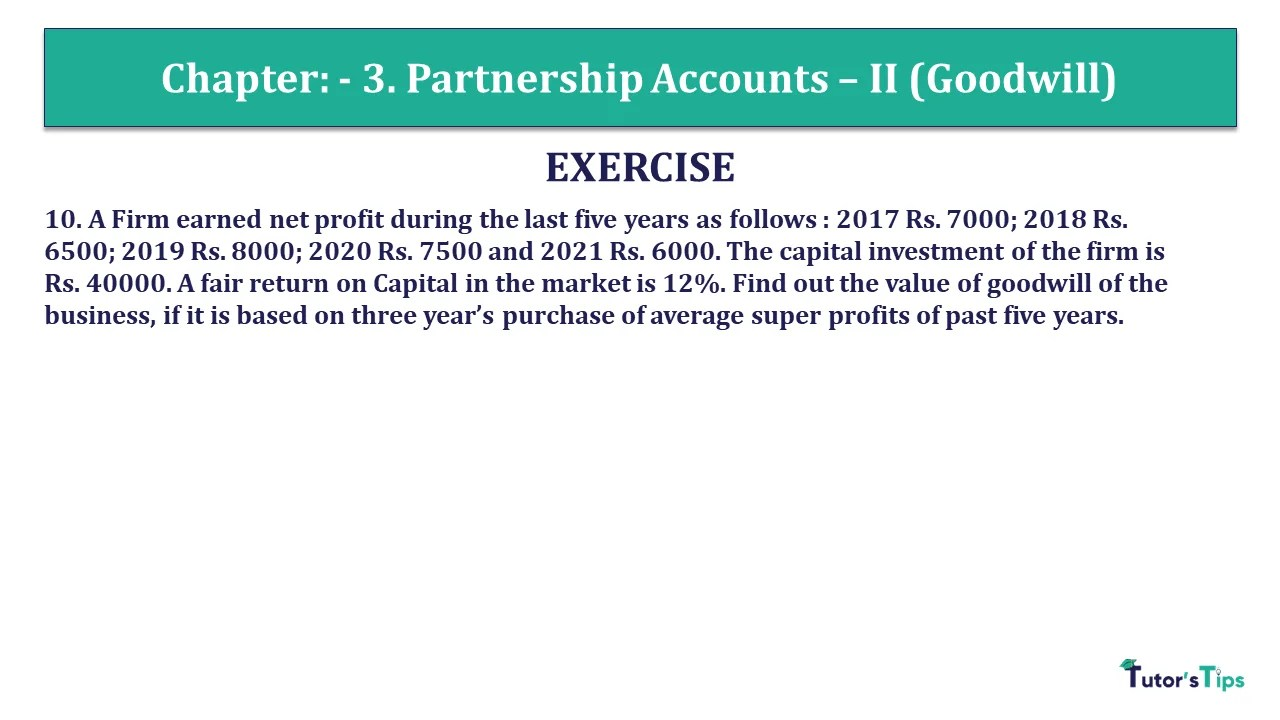# Question 10 Chapter 3 – Unimax Class 12 Part 1 – 2021Question 10 Chapter 3 – Unimax Class 12 Part 1

10. A Firm earned net profit during the last five years as follows : 2017 Rs. 7000; 2018 Rs. 6500; 2019 Rs. 8000; 2020 Rs. 7500 and 2021 Rs. 6000. The capital investment of the firm is Rs. 40000. A fair return on Capital in the market is 12%. Find out the value of goodwill of the business, if it is based on three year’s purchase of average super profits of past five years.

## The solution of Question 10 Chapter 3 – Unimax Class 12 Part 1:

Total Profits = Rs. 7000 + Rs. 6500 + Rs. 8000 + Rs. 7500 + Rs. 6000= Rs. 35000
Average Profits = Total Profits/Number of years
Normal Profits = Capital employed X Normal rate of return
= Rs. 40000 X 12/100
= Rs. 4800
Super Profits = Average Profits – Normal Profits
= Rs. 7000 – Rs. 4800
= Rs. 2200
Goodwill = Super Profits X Number of years of purchase of super profits
= Rs. 2200 X 3 years
= Rs. 6600

What is Partnership – Meaning and its Types

T.S. Grewal’s Double Entry Book Keeping +2 (Vol. I: Accounting for Not-for-Profit Organizations and Partnership Firms)